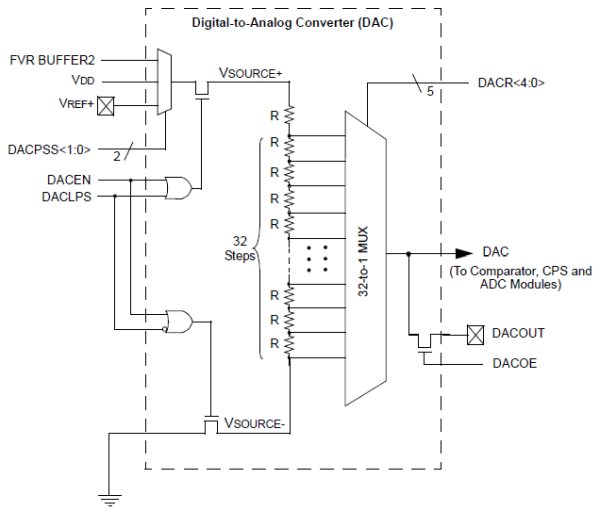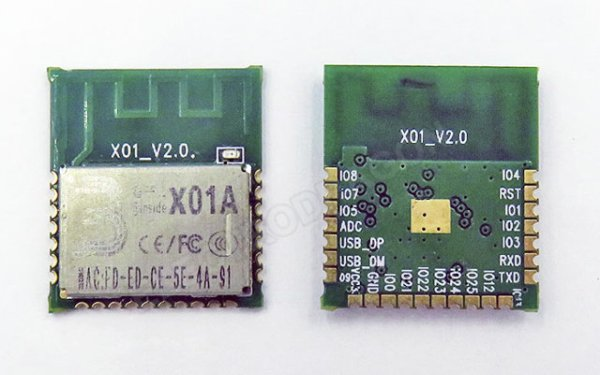PIC12F1822 DAC Module

This small post shows how to start using PIC12F1822 DAC (Digital-to Analog Converter) module.
PIC12F1822 microcontroller has 1 DAC module. The DAC can be used to supply analog voltage on RA0 pin with 32 selectable output levels.The input of the DAC can be connected to:

• External VREF pins
• VDD supply voltage
• FVR (Fixed Voltage Reference)

DAC Block diagram is shown below:

With CCS PIC C compiler we can initialize the DAC module using the following command:
setup_dac(int8 mode);
Where mode can one of the following modes:
DAC_OFF  0                                            // DAC disabled
DAC_VSS_VDD                                      // Negative source is VSS and positive source is VDD
DAC_VSS_VREF                                    // Negative source is VSS and positive source is Vref+ pin
DAC_VSS_FVR                                      // Negative source is VSS and positive source is FVR (Fixed Voltage Reference)
The DAC output can be enabled using the following line which or’ed with above in setup_dac() using |.
DAC_OUTPUT                                       // Enable ADC output on RA0 pin.
Controlling the output of the DAC is also easy with CCS PIC C compiler and for that we have to use the following command:
dac_write(int8 value);
Where value should be a 5-bit number which varies between 0 and 31.
The DAC output voltage is determined by the following equation assuming that the DAC is enabled (DACEN bit is 1):

The DAC 32 levels are set with DACR[4:0] bits which is done using dac_write() command.
Assume that we’ve PIC12F1822 microcontroller with +5V power supply, the DAC is configured with VSS and VDD as negative and positive sources and the DAC output is enabled.
Minimum Vout voltage when DACR[4 : 0] = 0b00000 equals to 0V.
Maximum Vout voltage when DACR[4 : 0] = 0b11111 equals to 4.84V
Writing DACR[4 : 0] = 0b00000 is done using: dac_write(0);
And DACR[4 : 0] = 0b11111 is dac_write(31);

PIC12F1822 DAC Module Example:
This is a small example for the DAC module. Example circuit schematic is shown below.

READ  TPS65262 – Triple Synchronous Step-Down Converter with 200mA/100mA Dual LDOs

Read more: PIC12F1822 DAC Module